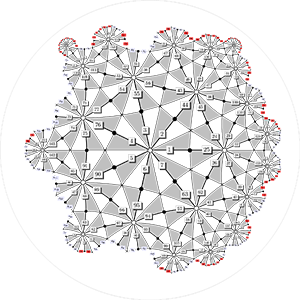This website uses features that are not well-supported by your browser. Please consider upgrading to a browser and version that fully supports CSS Grid and the CSS Flexible Box Layout Module.
Algebra and Number TheoryA dessin d'enfant is a special type of graph embedded on a Riemann surface whose geometry encodes number theoretic information. Here is an example of a dessin d'enfant conformally drawn on a fundamental domain for the Klein quartic defined by the equation $x^3y + y^3z + z^3x = 0$. Roughly speaking, number theory is the study of the integers. Carl Friedrich Gauss is said to have claimed: "Mathematics is the queen of the sciences and number theory is the queen of mathematics." Number theorists are interested in topics like the distribution of prime numbers, the solutions to systems of polynomial equations with integer coefficients, the structure of symmetry groups of the roots of a polynomial, and the very deep generalizations of these topics. Many problems in number theory are simple to state but have surprising solutions that draw broadly from all areas of mathematics, and conjectures in number theory have stimulated major advances in other fields. Number theory is both beautiful in its abstraction and useful in practice: the foundation of modern cryptographic systems rely crucially on the difficulty of certain number theoretic problems.

### Members

Asher Auel
Algebraic geometry; Number theory; Associative rings and algebras
Carl Pomerance
Number theory
Tom Shemanske
Number theory
John Voight
Number theory; Arithmetic geometry; Algebraic computing